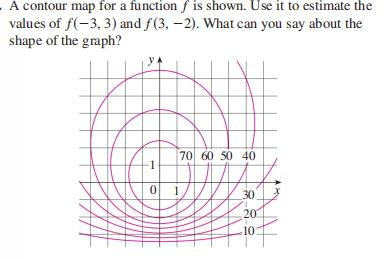### ¿Todavía tienes preguntas de matemáticas?

Pregunte a nuestros tutores expertos
Algebra
PreguntaA contour map for a function $$f$$ is shown. Use it to estimate the values of $$f ( - 3,3 )$$ and $$f ( 3 , - 2 )$$ . What can you say about the shape of the graph?

$$\approx 56 , \approx 35$$